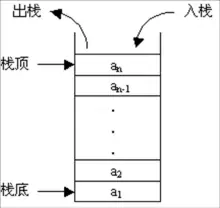03/11 18:37

# 栈## 栈的常用操作

type Stack Interface {
Push(x interface{})
Pop() interface{}
Size() int
Empty() bool
}

func StackDemo(sta Stack) {
sta.size()//判断栈的大小   0
sta.empty()//判断栈是否为空 true
sta.push(1) //入栈
sta.size()//判断栈的大小  1
sta.empty()//判断栈是否为空 false
sta.pop() //出栈
sta.size()//判断栈的大小  0
sta.empty()//判断栈是否为空  true
}


## 常见应用

### 进制转换

func MulBase(num int, base int) string {
//go里面用切片模拟栈即可（操作最后一个元素）
var stack []uint8
for {
if num <= 0 {
break
}
v := uint8(num % base)
if v > 9 {
v = v + 55
}else{
v = v + 48
}
//讲结果入栈
stack = append(stack, v)
num = int(math.Floor(float64(num/base)))
}
l := len(stack)
var sb []uint8
for i:=l-1;i>-1;i-- {
//从栈顶出栈（这里用数组模拟）
sb = append(sb, stack[i])
}
return string(sb[:])
}


### 回文判断

function IsPalindrome(word string) bool{
var stack []byte
for i:=0;i<len(word);i++{
stack = apped(stack, word[i])
}

return string(stack[:]) == word
}


### leetcode 20

leetCode 20:给定一个只包括 '('，')'，'{'，'}'，'['，']' 的字符串，判断字符串是否有效。

func isValid(s string) bool {
var bt []byte
for i := 0; i < len(s); i++ {
if s[i] == '(' || s[i] == '{' || s[i] == '[' {
bt = append(bt, s[i])
} else {
l := len(bt)
if l < 1 {
return false
}
check := false
if s[i] == ')' {
if bt[l-1] == '(' {
bt = bt[:l-1]
check = true
}
} else {
if bt[l-1]+2 == s[i] {
bt = bt[:l-1]
check = true

}
}
if !check {
return false
}
}

}
if len(bt) > 0 {
return false
}
return true
}


func isValid(s string) bool {
n := len(s)
if n % 2 == 1 {
return false
}
pairs := map[byte]byte{
')': '(',
']': '[',
'}': '{',
}
stack := []byte{}
for i := 0; i < n; i++ {
if pairs[s[i]] > 0 {
if len(stack) == 0 || stack[len(stack)-1] != pairs[s[i]] {
return false
}
stack = stack[:len(stack)-1]
} else {
stack = append(stack, s[i])
}
}
return len(stack) == 0
}


### 基本计算器,leetcode 224

输入：s = "1 + 1"



输入：s = " 2-1 + 2 "



输入：s = "(1+(4+5+2)-3)+(6+8)"


func calculate(s string) int {
var ans int
ops := []int{1}
sign := 1     // +,-     1 => + -1 => -
n := len(s)
for i := 0; i < n; {
switch s[i] {
case ' ':  //空格跳过
i++
case '+':
sign = ops[len(ops)-1]  //操作符为最后一个数字的符号
i++
case '-':
sign = -ops[len(ops)-1] //操作符为最后一个数字的符号取反
i++
case '(':
ops = append(ops, sign) //遇到括号，将最后一个符号入栈   LIFO
i++
case ')':
ops = ops[:len(ops)-1]  //遇到括号结束， 移除最后一个标志符号
i++
default:
num := 0
// int(s[i]-'0')  ascii 计算 -0 就为数字本身的值 技巧 get
// 和 int(s[i] -  48) 一样
//如果当前迭代器是0~9范围内的数字 则进行计算
//为什么再写一次循环在switch内呢？
//因为自增的i是不变的，所以时间复杂度还是o(n)
//但是 如果i++写在外部， 会增加多次的switch操作
for ; i < n && '0' <= s[i] && s[i] <= '9'; i++ {
// num = num*10 是最进位用 从示例来说， 是不需要的，但是题目并没有说数字仅仅是个位数，因此需要用到
num = num*10 + int(s[i]-'0')
}
ans += sign * num
}
}
return ans
}


### 计算器||， leetcode227

输入：s = "3+2*2"



输入：s = " 3/2 "



输入：s = " 3+5 / 2 "


func calculate(s string) int {
var stack []int
var res int
var preOp = '+' //第一个操作符肯定是作为+  比如 "3" => 3
num := 0        //上一次的数字，也就是符号之前的
for i, ch := range s {
isNum := ch > 47 && ch < 58
if isNum { //如果是数字则计算出当前数额
num = num*10 + int(ch-'0')
}

if i != len(s)-1 { //如果不是最后一个字符
if isNum || ch == ' ' { //如果是数字 或者是空格  不处理
continue
}
}
//根据上一个操作符计算数据，可以把整个计算器看成  （表达式） + （表达式） + （表达式）
// 将表达式结果入栈  最后将栈元素相加就行
switch preOp {
case '+':
stack = append(stack, num) // +n
case '-':
stack = append(stack, -num) //计算当前表达式 -n
case '*':
stack[len(stack)-1] *= num //计算当前表达式  m*n
case '/':
stack[len(stack)-1] /= num //计算当前表达式  m/n
}
preOp = ch // 上一次的符号决定当前数字的计算逻辑
num = 0

}
for _, v := range stack {
res += v
}

return res
}


0
0 收藏

### 作者的其它热门文章0 评论
0 收藏
0